MORE IN Elements of Civil Engg. & Engg. Mechanics
VTU First Year Engineering (P Cycle) (Semester 2)
Elements of Civil Engg. & Engg. Mechanics
December 2015
Total marks: --
Total time: --
INSTRUCTIONS
(1) Assume appropriate data and state your reasons
(2) Marks are given to the right of every question
(3) Draw neat diagrams wherever necessary

1 (a) Briefly explain the scope of any three fields of Civil Engineering.
10 M
1 (b) Write the classification of roads and comparison of flexible and rigid pavements.
10 M

2 (a) Define Force and write the characteristics of forces with examples.
8 M
2 (b) Determine angle θ (0≤θ≤180°) for the force F=200kN shown in fig. Q2(b), so that produces: i) Maximum moment about 'A' and ii) Minimum moment about 'A'.
Determine maximum and minimum moments.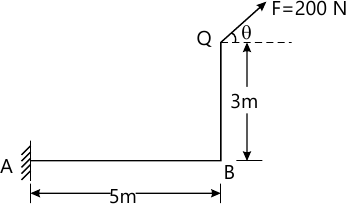8 M
2 (c) State and explain principle of transmissibility of a force.
4 M

3 (a) State and prove that parallelogram of forces.
8 M
3 (b) Define Resolution of a force with diagram.
4 M
3 (c) A barge is pulled by two tug boats as shown in fig Q3(c). If the resultant of the forces exerted by the tug boats is 5KN force directed along the axis of the barge. Determine the tension in each of the ropes knowing that α=457deg;.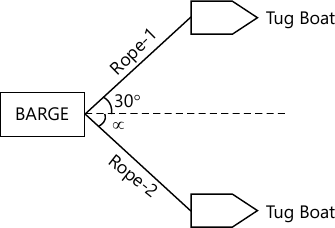8 M

4 (a) Define Moments and write the analytical conditions of equilibrium for a coplanar nor concurrent force system.
5 M
4 (b) Force system shown in fig Q4(b) has a resultant of 2kN acting up along Y-axis compute the force 'F' and its direction '&theta' with the horizontal, to give this resultant.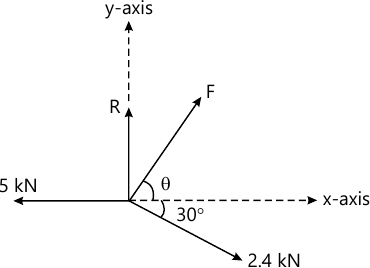7 M
4 (c) Determine the resultant of forces acting on cross section of dam shown in fig. Q4(c) and locate its intersection with the base AB. For good design, this intersection should occur within the middle third of the base. Does it?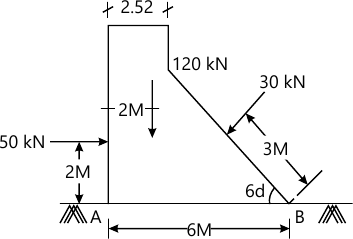8 M

5 (a) Determine the values of W1 and W2 shown in fig Q5(a). So that the part BC of the string is horizontal. Calculate the tension in the parts AB, BC, CD and DE. Also calculate the pressure on the frictionless pulley at D.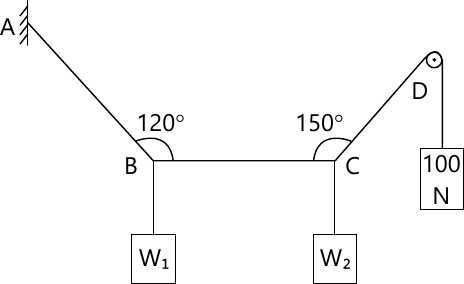10 M
5 (b) The cylinders P and Q weigh 20kN and 10kN. The corresponding diameters are 2.8m and 1.6m and are shown in fig.Q5(b). Determine the reactions of A, B, C and D.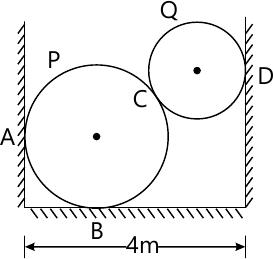10 M

6 (a) Define Equilibrium and Equilibriant, with neat diagram.
4 M
6 (b) Explain: i) Coefficient of friction
ii) Angle of Repose
iii) Cone of friction, with neat diagrams.
6 M
6 (c) Two blocks are placed as shown in fig. Q6(c). Weight of block A is 5kN and of block B is 4kN. The coefficient of friction between all surface in contact is 0.2. Find the effort required to start moving block B and also the tension in the cable.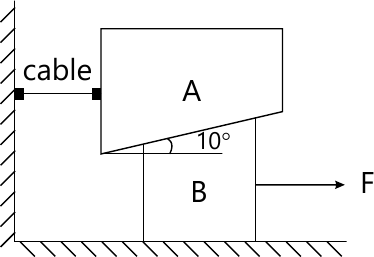10 M

7 (a) Determine the centroid of a right angle triangle of base 'b' and height 'h' from first principles.
8 M
7 (b) Determine the polar radius of gyration about the centroidal axes of the section shown in fig. Q7(b).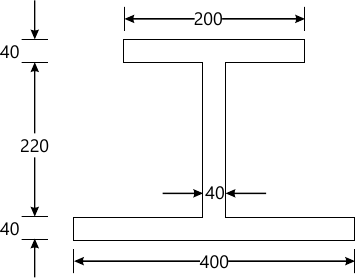12 M

8 (a) Derive the expression for the M.I. of a semicircular lamina of radius (r) about its centroidal axis parallel to the diameter.
8 M
8 (b) Determine the centroid of lamina shown in fig. Q8(b) and mark the centroid.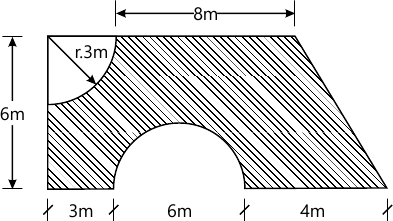12 M

9 (a) Define i) Rectilinear motion
ii) Curvilinear motion with example.
6 M
9 (b) Explain the following with sketch:
i) Angle projection
ii) Time of flight
iii) Range.
6 M
9 (c) A stone is thrown vertically upwards and returns to the earth in 10S. What was its initial velocity and how high did it go?
8 M

10 (a) Derive an expression for maximum height of a projectile on a horizontal plane.
8 M
10 (b) A cricket ball thrown by a player from a height of 2.0m above the horizontal ground at an angle of 30° to the horizontal and with a velocity of 12m/s. The ball hits the wicket at a height of 0.6m above the ground. How far is the player from the wicket?
12 M

More question papers from Elements of Civil Engg. & Engg. Mechanics